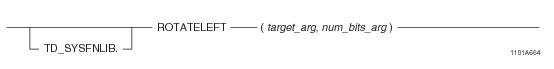## SQL Functions, Operators, Expressions, and Predicates

Product
Release Number
15.00
Language
English (United States)
Last Update
2018-09-24
dita:id
B035-1145
lifecycle
previous
Product Category

## ROTATELEFT

### Purpose

Returns the expression target_arg rotated by the specified number of bits (num_bits_arg) to the right, with the most significant bits wrapping around to the left.

### Syntaxwhere:

 Syntax element… Specifies… TD_SYSFNLIB the name of the database where the function is located. target_arg a numeric or variable expression. num_bits_arg an integer expression indicating the number of bit positions to rotate.

### ANSI Compliance

This is a Teradata extension to the ANSI SQL:2011 standard.

### Description

 IF... THEN the function... num_bits_arg is equal to zero returns target_arg unchanged. num_bits_arg is negative rotates the bits to the right instead of the left. target_arg and/or num_bits_arg are NULL returns NULL. num_bits_arg is larger than the size of target_arg rotates (num_bits_arg MOD sizeof(target_arg)) bits. The scope of the rotation operation is bounded by the size of the target_arg expression.

Note: When operating against an integer value (BYTEINT, SMALLINT, INTEGER, or BIGINT), rotating a bit into the most significant position will result in the integer becoming negative. This is because all integers in Teradata Database are signed integers.

### Argument Types and Rules

ROTATELEFT is an overloaded scalar function. It is defined with the following parameter data types for the following (target_arg, num_bits_arg) input combinations:

 target_arg type num_bits_arg type BYTEINT INTEGER SMALLINT INTEGER INTEGER INTEGER BIGINT INTEGER VARBYTE(n) INTEGER

The maximum supported size (n) for VARBYTE is 8192 bytes.

All expressions passed to this function must either match these declared data types or can be converted to these types using the implicit data type conversion rules that apply to UDFs.

Note: The UDF implicit type conversion rules are more restrictive than the implicit type conversion rules normally used by Teradata Database. If any argument cannot be converted to one of the declared data types by following UDF implicit conversion rules, it must be explicitly cast. For details, see “Compatible Types” and “Parameter Types in Overloaded Functions” in SQL External Routine Programming.

If any argument cannot be converted to one of the declared data types, an error is returned indicating that no function exists that matches the DML UDF expression submitted.

### Result Type and Attributes

The result data type depends on the data type of the target_arg input argument that is passed to the function as shown in the following table:

 IF the data type of target_arg is... THEN the result type is... AND the result format is the default format for... BYTEINT BYTEINT BYTEINT SMALLINT SMALLINT SMALLINT INTEGER INTEGER INTEGER BIGINT BIGINT BIGINT VARBYTE(n) VARBYTE(n) VARBYTE(n)

The maximum supported size (n) for VARBYTE is 8192 bytes.

The default title for ROTATELEFT is: ROTATELEFT(target_arg, num_bits_arg).

For information on default data type formats, see SQL Data Types and Literals.

### Example

In the following query, the input argument 16 has a data type of BYTEINT and a binary representation of 00010000. When this value is rotated left by two bits, the result in binary is 01000000. This value translates to a BYTEINT value of 64, which is the result returned by the query.

`   SELECT ROTATELEFT(16,2);`

### Example

In the following query, the input argument 64 has a data type of BYTEINT and a binary representation of 01000000. When this value is rotated left by three bits, the result in binary is 00000010. This value translates to a BYTEINT value of 2, which is the result returned by the query.

`   SELECT ROTATELEFT(64,3);`